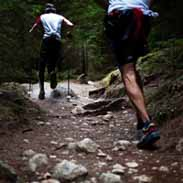# 5th Grade Science: FOSS VariableDavid Dunn
question

Variable

A factor that can be changed in a experiment and might affect the outcome
question

Standard Cycle

question

Pendulum

A mass hanging from a fixed point that is free to swing to and fro
question

System

A set of objects that work together
question

Mass

The amount of matter an object contains
question

Lifeboat

A boat used to carry passengers away from sinking ships
question

Two-Coordinate Graph

A graph that shows the outcome of a series of experience when a variable is changed by steps
question

Controlled Experiment

An experiment which one, and only one, variable is changed in order to test its effect
question

Plane

An aircraft that is heavier than air which is kept aloft by upward thrust of air on its fixed wings and driven by propellers
question

X Axis

The horizontal axis in a plane coordinate system
question

Y Axis

The vertical axis in a plane coordinate system
question

Catapult

A lever arm that is loaded with energy. When the energy is released, any object resting on the end of the lever is hurled into the air
question

Controlled Variable

What is kept the same in a controlled experiment to ensure a \”fair test\”
question

Independent Variable

The variable that is changed in an experiment
question

Dependent Variable

The variable that is measured in an experiment
question

How many trials should a good scientist conduct?

3 or more
question

What was the relationship we discovered between the length of our swingers and the amount of swings?

The longer the swinger the fewer the swings. The shorter the swinger the more swings
question

On what axis is the independent variable graphed?

The X axis
question

On what axis is the dependent variable graphed?

The Y Axis
question

What were the parts of our swinger system?

String, penny (mass), tape, paper clip, and a pencil
question

How can you tell if you need to retest the outcome of a trial after graphing your data?

The outcome does not match or is too far away from our line of best fit
question

Cycle

Any pattern that repeats itself
question

Prediction

An estimation based on information or experience
question

In our swingers experiment, what was/were the independent variable(s)?

The length of the string
question

In our swingers experiment, what was/were the dependent variable(s)?

The number of cycles in 15 seconds
question

In our swingers experiment, what was/were the controlled variables?

The mass of the bob and the release position
question

Standard

A procedure that has been established to compare future experiments with
question

True or False, this is a controlled experiment: John wanted to test how fast different batteries ran out of power. He put some batteries in his toys, then he played with them. His radio controlled can batteries failed before his calculator did so he concluded those batteries had less power.

False
question

Why is this not a controlled experiment? John wanted to test how fast different batteries ran out of power. He put some batteries in his toys, then he played with them. His radio controlled can batteries failed before his calculator did so he concluded those batteries had less power.

He tested two different toys, each could have used different amounts of batteries, the calculator could use less power. He only tested once. He didn’t control his variables
question

What was the relationship we discovered between the capacity of our lifeboats and the passengers it could hold?

The greater the capacity the more passengers it could hold
question

What was the relationship we discovered between the distance our foss plane travelled and the passengers (mass) it could carry?

The more passengers (mass) you have the shorter the distance your plane will travel
question

What was the relationship we discovered between the length of our swingers and the amount of swings?

The longer the swinger the fewer the swings. The shorter the swinger the more swings
question

What was the relationship we discovered between distance our foss plane travelled and the angle of the flight line?

The higher the angle the shorter the distance the plane traveled
question

What changes could you make to our flipper system to make our cork go higher?

Choose the cork with the least mass, put the cork near the end of the flipper, push the flipper stick down all of the way, and push the flipper to the 1 cm mark
question

What changes could you make to our flipper system to make the cork travel farther?

Choose the cork with the least mass, put the cork near the end of the flipper stick, only push the flipper stick down part way, move the flipper to 3 cm mark
question

What were the parts of our swinger system?

String, penny (mass), tape, paper clip, and a pencil
question

What were the parts of our flipper (catapult) system?

Cork (Projectile), Base, Angle Adjuster, Popsicle Stick (arm)
question

What were the parts of our lifeboat system?

Paper cup, passengers (mass), water, and basin
question

What were the parts of our foss plane system?

craft sticks, rubber band, propeller, straws, plastic hooks, tape, the flight line, measurer
question

What was the relationship between the angle of the catapult base and the distance travelled by the ball?

The higher the angle the farther the ball travelled
question

What can you use to predict the results of an experiment?

A two coordinate graph or another form of a graph
question

What are the three types of graphs we used during our investigations?

concrete, picture, two coordinate graphs
question

Capacity